# The decomposition of N, O, can be described by the equation 2N,0; (soln) + 4NO, (soln)...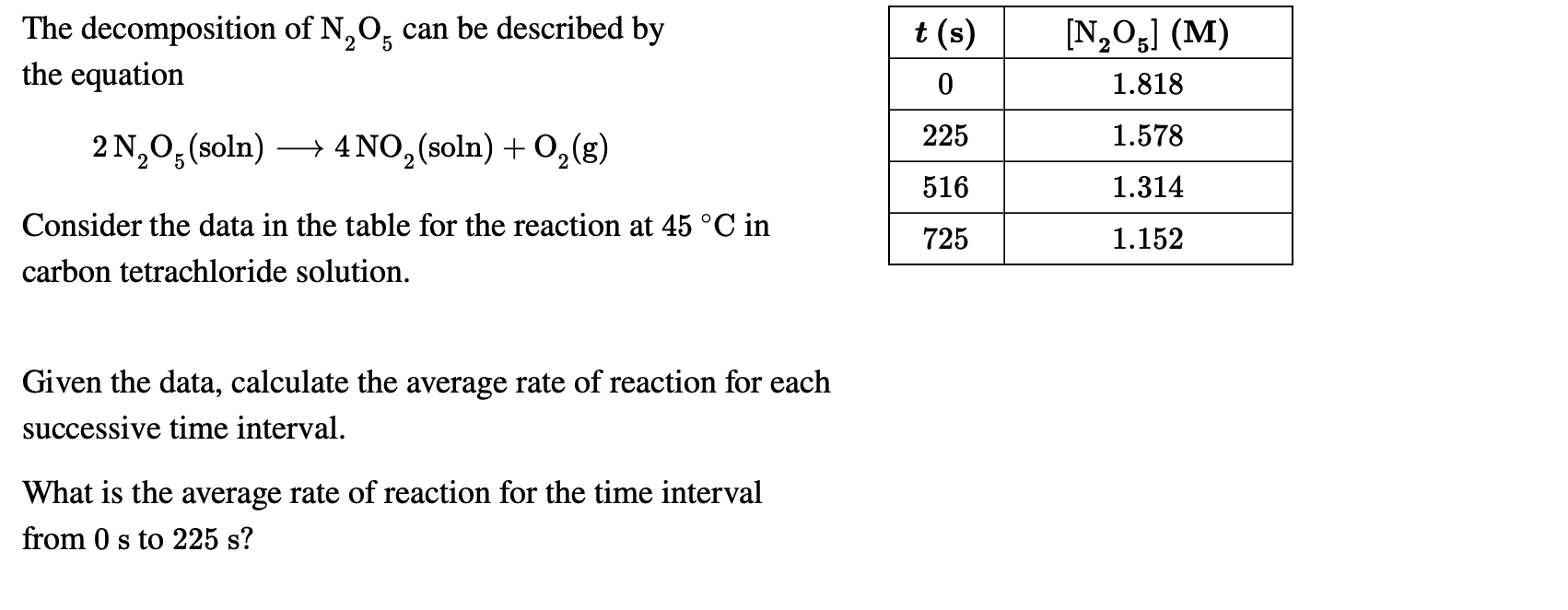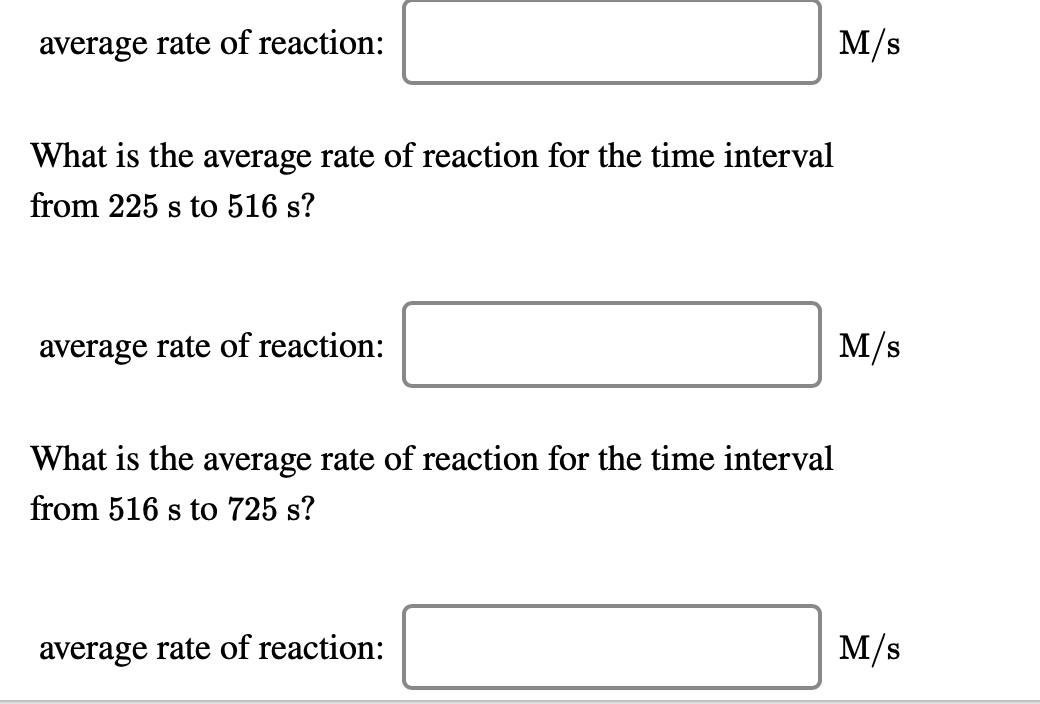The decomposition of N, O, can be described by the equation 2N,0; (soln) + 4NO, (soln) + O2(g) t(s) O 225 516 | 725 [N,03] (M) 1.818 1.578 1.314 1.152 Consider the data in the table for the reaction at 45 °C in carbon tetrachloride solution. Given the data, calculate the average rate of reaction for each successive time interval. What is the average rate of reaction for the time interval from 0 s to 225 s?
average rate of reaction: M/s What is the average rate of reaction for the time interval from 225 s to 516 s? average rate of reaction: M/s What is the average rate of reaction for the time interval from 516 s to 725 s? average rate of reaction:

The average reaction speed in each section is calculated:

i) Rate = (1.818 - 1.578) / (225 - 0) = 0.0011 M / s

ii) Rate = (1,578 - 1,314) / (516 - 225) = 0.0009 M / s

iii) Rate = (1,314 - 1,152) / (725 - 516) = 0.0008 M / s

If you liked the answer, please rate it positively, you would help me a lot, thanks.

#### Earn Coin

Coins can be redeemed for fabulous gifts.

Similar Homework Help Questions
• ### The decomposition of N,Os can be described by the equation t(s) 0 2N20: (soln) 4NO2 (soln)...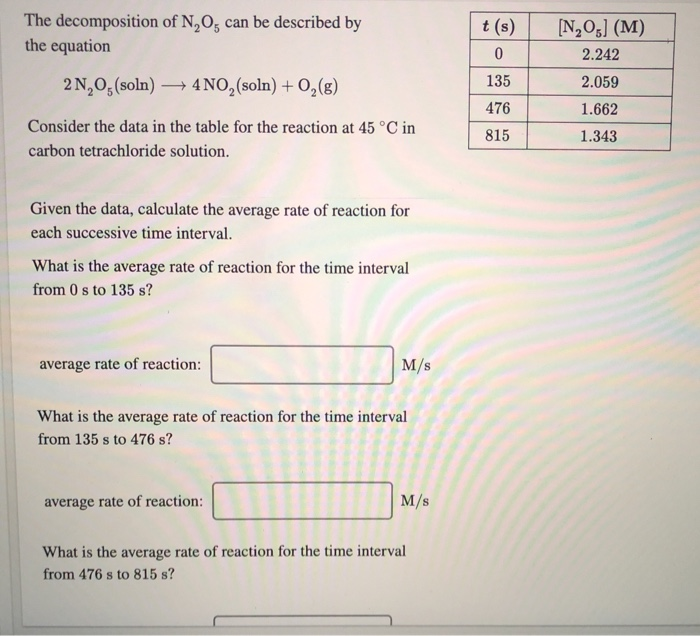The decomposition of N,Os can be described by the equation t(s) 0 2N20: (soln) 4NO2 (soln) + 02 (8) 135 476 [N, 05] (M) 2.242 2.059 1.662 1.343 Consider the data in the table for the reaction at 45 °C in carbon tetrachloride solution. 815 Given the data, calculate the average rate of reaction for each successive time interval. What is the average rate of reaction for the time interval from 0 s to 135 s? average rate of reaction:...

• ### The decomposition of N, O, can be described by the equation /(s) [N,931(M) 1.871 0 2N,...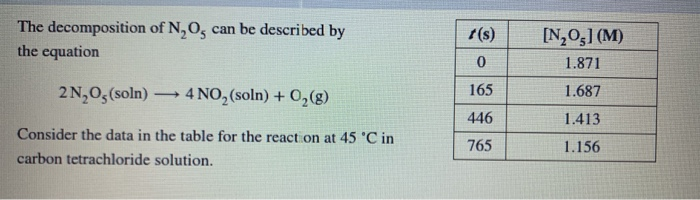The decomposition of N, O, can be described by the equation /(s) [N,931(M) 1.871 0 2N, O (soln) — 4 NO, (soln) + O2(g) 165 1.687 446 1.413 Consider the data in the table for the reaction at 45 'C in carbon tetrachloride solution. 765 1.156 Given the data, calculate the average rate of reaction for each successive time interval. What is the average rate of reaction for the time interval from Os to 165 s? average rate of reaction:...

• ### Question 5 of 18 > The decomposition of N, O, can be described by the equation...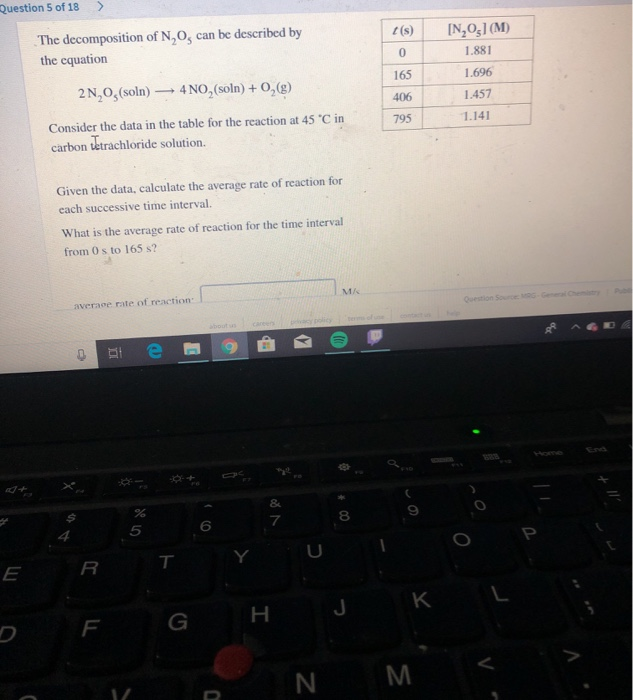Question 5 of 18 > The decomposition of N, O, can be described by the equation t(s) 0 165 406 795 [N,O31(M) 1.881 1.696 1.457 1.141 2N, O,(soln) -4NO, (soln) + 0, (g) Consider the data in the table for the reaction at 45 °C in carbon tetrachloride solution. Given the data, calculate the average rate of reaction for each successive time interval. What is the average rate of reaction for the time interval from 0 to 165 s? average...

• ### t(s) The decomposition of N2O5 can be described by the equation [N2O3] (M) 1.798 0 225...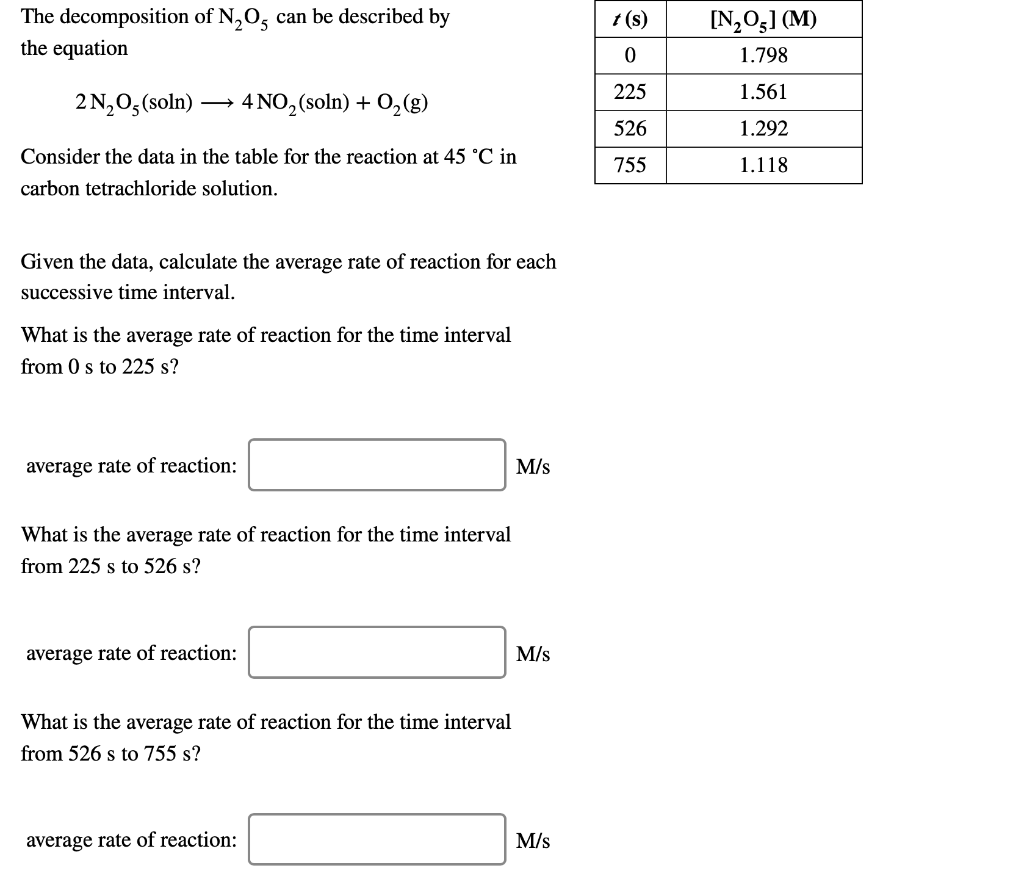t(s) The decomposition of N2O5 can be described by the equation [N2O3] (M) 1.798 0 225 1.561 2N203 (soln) 4NO2 (soln) + O2(g) 526 1.292 Consider the data in the table for the reaction at 45 °C in carbon tetrachloride solution. 755 1.118 Given the data, calculate the average rate of reaction for each successive time interval. What is the average rate of reaction for the time interval from 0 s to 225 s? average rate of reaction: M/s What...

• ### The decomposition of N2O5N2O5 can be described by the equation 2N2O5(soln)⟶4NO2(soln)+O2(g) Consider the data in the...

The decomposition of N2O5N2O5 can be described by the equation 2N2O5(soln)⟶4NO2(soln)+O2(g) Consider the data in the table for the reaction at 45 ∘C45 ∘C in carbon tetrachloride solution. ? (?)t (s) [N2O5] (?)[N2O5] (M) 0 2.540 205 2.233 476 1.883 765 1.570 Given the data, calculate the average rate of reaction for each successive time interval. What is the average rate of reaction for the time interval from 0 s0 s to 205 s? average rate of reaction: M/sM/s What...

• ### Help The decomposition of N can be described by the equation t (s) DN205 (M) 2.50...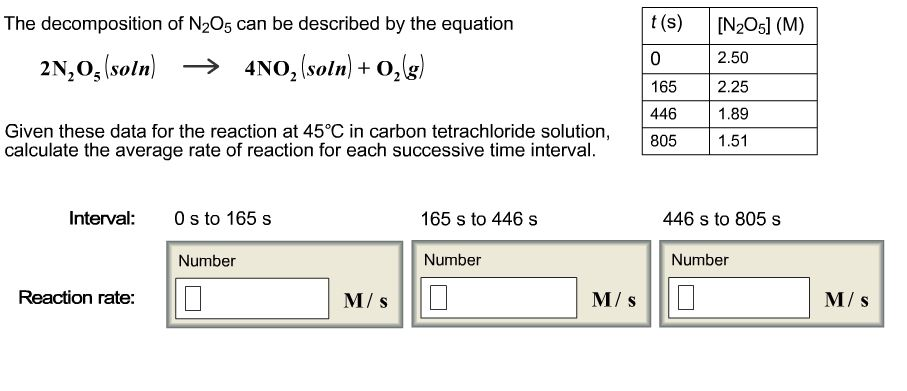Help The decomposition of N can be described by the equation t (s) DN205 (M) 2.50 2N,os (soln) -3 4NO2 (soln) 0, (g) 165 2.25 446 1.89 Given these data for the reaction at 45°C in carbon tetrachloride solution, 805 1.51 calculate the average rate of reaction for each successive time interval. L Interval 0 s to 165 s 165 s to 446 s 446 s to 805 s Number Number Reaction rate: D Number

• ### The decomposition of N2O5 can be described by the equation 2N_2O_5(soln) rightarrow 4N02(soln) + 0_2(g) Given...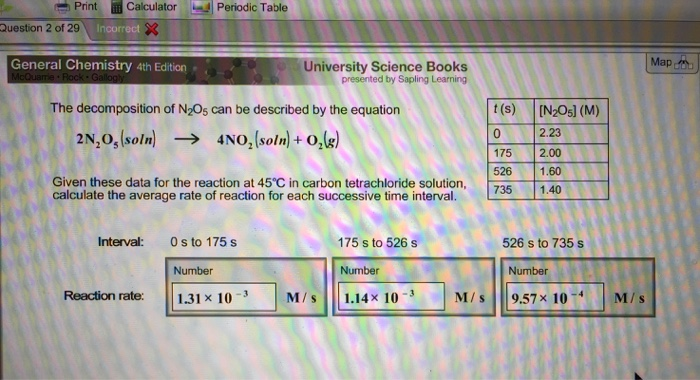The decomposition of N2O5 can be described by the equation 2N_2O_5(soln) rightarrow 4N02(soln) + 0_2(g) Given these data for the reaction at 45 degree C in carbon tetrachloride solution, calculate the average rate of reaction for each successive time interval.

• ### The decomposition of N20s can be described by the equation t (s) [N2Osl (M) 24,05(soln) 4NO2(soln)...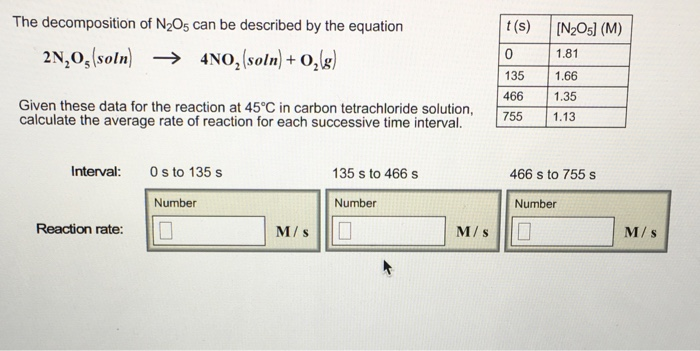The decomposition of N20s can be described by the equation t (s) [N2Osl (M) 24,05(soln) 4NO2(soln) +02(g) → 1.81 135 1.66 466 1.35 Given these data for the reaction at 45°C in carbon tetrachloride solution calculate the average rate of reaction for each successive time intervalL Interval: 0 s to 135 s 135 s to 466 s 466 s to 755 s Number Number Number Reaction rate: M/ s M/ s M/s

• ### The decomposition of N2O5 can be described by the equation. 2N2O5

The decomposition of N2O5 can be described by the equation. 2N2O5 (soln) --->  4NO2 (soln) + 2 (g) Given this data for the reaction at 45 degrees C in carbon tetrachloride solution, calculate the average rate for each successive time interval. t(s)                [N2O5] (M) 0                    2.10 195                1.86 556                1.48 825                1.25 Interval: 0 s to 195 s Reaction rate= _____M/s 195 s to 556 s Reaction rate= _____M/s 556 s to 825 s Reaction rate= _____M/s

• ### 1. What is the average rate of reaction for the time interval from 0 s0 s...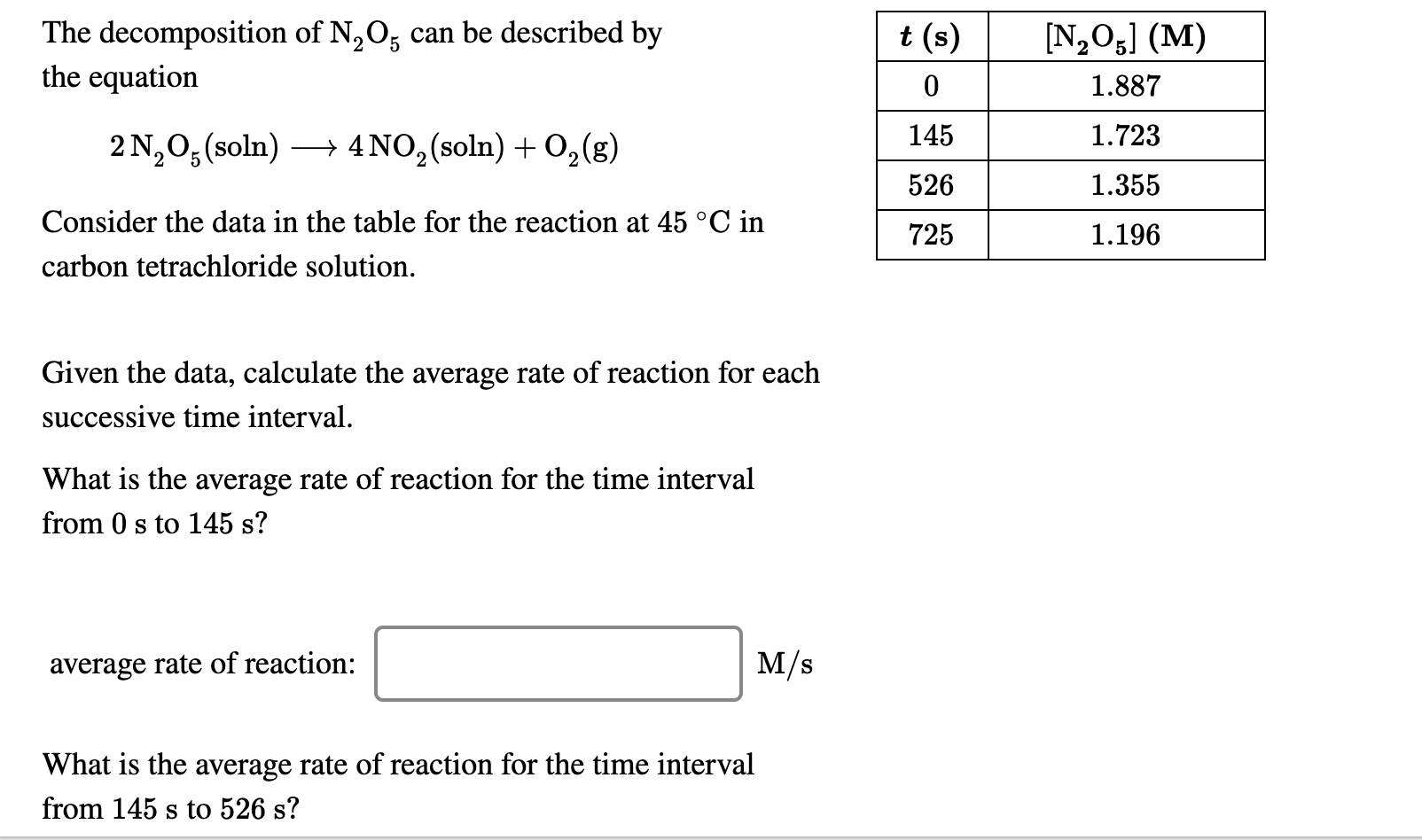1. What is the average rate of reaction for the time interval from 0 s0 s to 145 s? 2. What is the average rate of reaction for the time interval from 145 s145 s to 526 s? 3. What is the average rate of reaction for the time interval from 526 s526 s to 725 s? The decomposition of N20, can be described by the equation t(s) 0 145 526 [N,05] (M) 1.887 1.723 2 N20(soln) —+ 4NO2 (soln)...